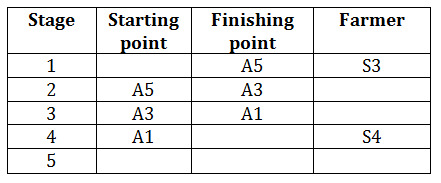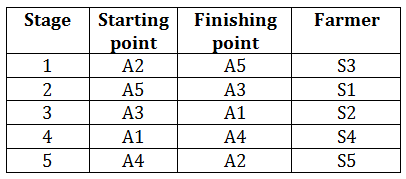### IBPS reasoning practice set: IBPS reasoning practice set that you should solve for exam

IBPS reasoning practice set consists of a variety of questions in the form of sets that are important from the exam point of view. These sets help you gain a better understanding of concepts, develop a broader perspective and work towards establishing better methods to solve problems. The main aim of the IBPS reasoning practice set is to help students shape their preparation and propel it in the right direction. Therefore, go through the concepts and get ready to solve these practice sets so that you make the most of it. Time yourself with realistic deadlines to solve each set and then refer solutions to find out the possibility of a better approach.

Directions for the question set:
A circular field, with inner radius of 10 meters and outer radius of 20 meters, was divided into five successive stages for ploughing. The ploughing of each stages was handed over to a different farmer.
1. Farmers are referred to by following symbols: S1, S2, S3, S4, S5.
2. The points between different stages of project are referred to by the following symbols: A1, A2, A3, A4, A5, not necessarily in the same order.
3. Farmer S4 was given the work of the fourth stage.
4. Stage 3 finished at point A1, and the work of which was not given to farmer S1.
5. Farmer S3 was given work of stage ending at point A5.
6. Farmer S5 was given the work of ploughing stage starting at point A4.
7. The stage from point A5 to point A3 was not the first stage.

Question 1: Which were the starting and the finish points of stage 2?
A. A2 and A5
B. A5 and A3
C. A3 and A1
D. A5 and A4
E. A3 and A2

Question 2: For which farmer was A2 a finishing point?
A. S1
B. S2
C. S3
D. S4
E. S5

Question 3: Which stage was ploughed by farmer S5?
A. First
B. Second
C. Third
D. Fourth
E. Fifth

Question 4: Which was the starting point for Farmer S3?
A. A2
B. A3
C. A4
D. A1
E. None of above

Question 5: Which was the finish point for farmer S2?
A. A1
B. A2
C. A3
D. A4
E. A5

### Answers and Explanations: Click the down arrow to expand

Common Solution for the Set: Stage 3 finished at point A1 and the work in the stage 4 was done by S4. After this we need to see the last clue which states that A3-A5 will be one starting and ending pair. Now to place this pair we only have 3 stages- 1st ,2nd or last. Since from clue 7, this pair will not be in the first place, so it can be in only the second or last stage. After this, focus on the implied clue from clue 5, which implies that A5 is the ending point of some stage where S3 was given the work. If we place A5-A3 in last stage then it will mean that A5 will be the ending point of stage 4, but this fourth stage already has S4, hence we cannot place S3 in this, so we will have to place A5-A3 in the second stage with S3 farmer working in it. Now the table looks like-Now focus on the remaining clues. We see that S5 was given the work of stage starting with A4 (Clue 6), hence we must find an empty position in the above table in the starting point column. We see that only 1st stage and 5th stage starting points are vacant. Now if we choose 1st position then we will not be able to place S5 in there (clue 6) as it already has S3 in there, hence A4 should be placed in last stage starting point with S5. Now we just have to decide the position of S1 & S2 farmer. Now see clue 4, it states that stage 3 will not have S1, hence 2nd stage will have S1 while 3rd stage will have S2. Completed table will look like-Answer 1: (B) A5 and A3 are the starting and finishing points of stage 3. The correct option is (B).

Answer 2: (E) For farmer S5, A2 was the finishing point. The correct option is (E).

Answer 3: (E) Stage 5 was ploughed by farmer S5. The correct option is (E).

Answer 4: (A) A2 was the starting point of Farmer S3. The correct option is (A).

Answer 5: (A) A1 was the finishing point for famer S2. The correct option is (A).

Extra tips for IBPS reasoning practice set:
• IBPS reasoning practice set should be timed with realistic deadlines resonating with the actual exam.
• Use option elimination method wherever applicable to save time while solving IBPS reasoning practice set.
• Score well in IBPS reasoning practice set by thoroughly covering the concepts and then jumping on to solve the sums. In this way you will make the most of these practice exercises.